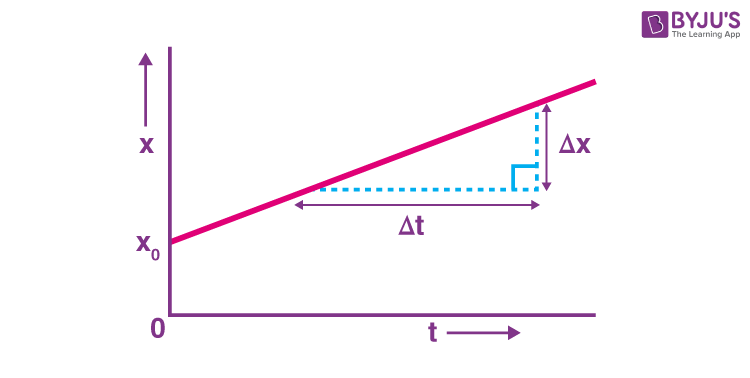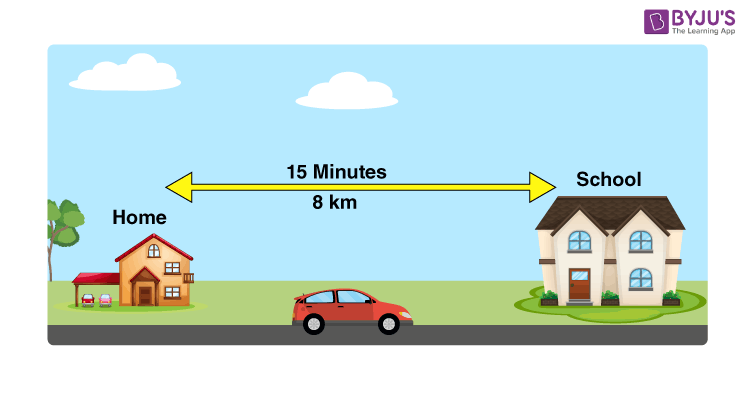# What Is Velocity?

The terms velocity and speed give us an idea of how fast or slow an object is moving. Quite often, we come across situations where we need to identify which of the two or more objects is moving faster. One can easily tell the faster of the two if they are moving in the same direction on the same road. However, if their direction of motion is in the opposite direction, then it is difficult to determine the fastest. In such cases, the concept of velocity is helpful. In this article, let us learn the velocity meaning, the unit of velocity, the example of velocity, and the difference between speed and velocity.

## Velocity Meaning

According to the velocity meaning, it can be defined as the rate of change of the object’s position with respect to a frame of reference and time. It might sound complicated, but velocity is basically speeding in a specific direction. It is a vector quantity, which means we need both magnitude (speed) and direction to define velocity. The SI unit of it is metre per second (ms-1). If there is a change in magnitude or the direction of the velocity of a body, then it is said to be accelerating.

### Initial and Final Velocity

Initial velocity describes how fast an object travels when gravity first applies force on the object. On the other hand, the final velocity is a vector quantity that measures the speed and direction of a moving body after it has reached its maximum acceleration.

#### How to find the final velocity?

Finding the final velocity is simple, with a few calculations and basic conceptual knowledge.

1. Determine the object’s original velocity by dividing the time it took for the object to travel a given distance by the total distance. In the equation V = d/t, V is the velocity, d is the distance, and t is the time.
2. Determine the object’s acceleration by dividing the object’s mass by force and multiply the answer by the time it took for it to accelerate. For example, if the object weighs 30 kg and has a force of 15 N applied to it, then the acceleration would be 4 m/s.
3. Add the quantity obtained from Step 1 and Step 2 to obtain the final velocity. For example, if your initial velocity was 3 m/s and your object acceleration is 4 m/s, your final velocity is 7 m/s (3 + 4 = 7).

## Constant Velocity

Motion with constant velocity is the simplest form of motion. We witness constant motion whenever an object slides over a horizontal, low-friction surface (when a puck slides over a hockey rink.)The above graph is a graph of displacement versus time for a body moving with constant velocity. The straight line in the graph can be algebraically represented as follows:

$$\begin{array}{l}x=x_0+vt\end{array}$$
In the equation, x0 is the displacement at time t, v is the constant velocity of the body
$$\begin{array}{l}v=\frac{dx}{dt}\end{array}$$

### Velocity Units

After learning the velocity meaning, let us know about the unit of velocity. The SI unit of velocity is m/s (m.s-1). Other units and dimensions of velocity are given in the table below.

 Unit of velocity Common symbols v, v, $$\begin{array}{l}\vec{v}\end{array}$$ SI unit m/s Other units mph, ft/s Dimension LT−1

## The below video provides the Top 10 NTSE Important Questions on Motion Class 9## Speed and Velocity

Speed and velocity can be a little confusing for most of us. Well, the difference between speed and velocity is that speed gives us an idea of how fast an object is moving, whereas velocity not only tells us its speed but also tells us the direction the body is moving in. We can define speed as a function of distance travelled, whereas velocity is a function of displacement. Instantaneous velocity is the velocity of a body at any given time. Average velocity is the total displacement by total time and is given by v = △x/△t where ∆x is the total displacement of the body and ∆t is the time. Average velocity is always less than or equal to that of average speed; this is because displacement can never be higher than the distance travelled, but the distance travelled can be higher than that of displacement.

### Example of Velocity

Let us learn the example of velocity after learning the meaning of velocity. To understand the concept of instantaneous velocity and average velocity, let’s take this example. Jewel goes to school in her dad’s car every morning. Her school is 8 km from her home, and she takes 15 mins to travel, but when she looks at the speedometer on the dashboard of the car, it shows a different reading all the time. So, now, how would she know her velocity?Well, the average velocity of Jewel’s car could be found by:

For convenience, we have considered the car to move in a straight line, and we will convert all the units of time to hours. Therefore, 15 mins = 1560 = 0.25 hours.

Average velocity, v = △x/△t

v = 8 km/0.25 hrs

v = 32 km/h

Now we see that even though the car may vary its speed if it covers the same amount of distance in the same amount of time, every time, its average velocity will remain the same.

## Difference between Speed and Velocity

The detailed comparison in the tabular format is given below.

 Velocity VS Speed SPEED VELOCITY Speed is the quantitative measure of how quickly something is moving. Velocity defines the direction of the movement of the body or the object. Speed is primarily a scalar quantity Velocity is essentially a vector quantity It is the rate of change of distance It is the rate of change of displacement Speed of an object moving can never be negative The velocity of a moving object can be zero. Speed is a prime indicator of the rapidity of the object. Velocity is the prime indicator of the position as well as the rapidity of the object. It can be defined as the distance covered by an object in unit time. Velocity can be defined as the displacement of the object in unit time.

#### Let’s summarize what we have learned:

• (a) This is a function of displacement.
• (b) We require both magnitude and direction to define velocity.
• (c) Instantaneous velocity is the velocity at any given moment of time, whereas average velocity is the total displacement divided by total time.
• (d) A changing velocity indicates acceleration.

## Watch the video below and learn about angular velocity along with velocity meaning## Frequently Asked Questions – FAQs

Q1

### Can vector quantities be added algebraically?

Vector quantities cannot be added algebraically because they have directions along with magnitude. And directions cannot be added algebraically.

Q2

### Give a general equation of velocity for a particle moving with velocity v such that $$\begin{array}{l}\vec{v} = k(y\hat{i}+x\hat{j})\end{array}$$ where k is constant.

The general of a particle moving with velocity

$$\begin{array}{l}\vec{v} = k(y\hat{i}+x\hat{j})\end{array}$$
is given as y2 = x2 + constant

Q3

### What is instantaneous speed?

Instantaneous speed is defined as the speed of an object at a specific moment in time.

Q4

### What happens to the velocity and acceleration when a ball is thrown in the air?

Velocity and acceleration both increase when the ball is thrown in the air.

Q5

### What is the purpose of the velocity-time graph?

The Velocity-time graph is used to explain the constant acceleration of an object.

## Watch the video and solve important questions in the chapter Motion Class 9Hope you have understood the velocity meaning, unit of velocity, constant velocity and the difference between speed and velocity in brief. Stay tuned with BYJU’S – The learning App to know more.